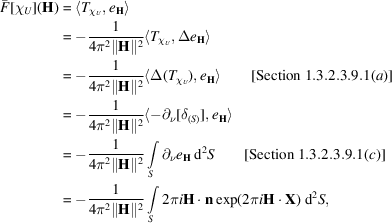International
Tables for
Crystallography
Volume B
Reciprocal space
Edited by U. Shmueli

International Tables for Crystallography (2010). Vol. B, ch. 1.3, p. 93   | 1 | 2 |

Section 1.3.4.4.3.5. Molecular-envelope transforms via Green's theorem

G. Bricognea

aGlobal Phasing Ltd, Sheraton House, Suites 14–16, Castle Park, Cambridge CB3 0AX, England, and LURE, Bâtiment 209D, Université Paris-Sud, 91405 Orsay, France

1.3.4.4.3.5. Molecular-envelope transforms via Green's theorem

| top | pdf |

Green's theorem stated in terms of distributions (Section 1.3.2.3.9.1) is particularly well suited to the calculation of the Fourier transformsof indicator functions. Let f be the indicator functionand let S be the boundary of U (assumed to be a smooth surface). The jumpin the value of f across S along the outer normal vector is, the jumpin the normal derivative of f across S is, and the Laplacian of f as a function is (almost everywhere) 0 so that. Green's theorem then reads:The functionsatisfies the identity. Therefore, in Cartesian coordinates:i.e.where n is the outer normal to S. This formula was used by von Laue (1936)for a different purpose, namely to calculate the transforms of crystal shapes (see also Ewald, 1940). If the surface S is given by a triangulation, the surface integral becomes a sum over all faces, since n is constant on each face. If U is a solid sphere with radius R, an integration by parts gives immediately:References

Ewald, P. P. (1940). X-ray diffraction by finite and imperfect crystal lattices. Proc. Phys. Soc. London, 52, 167–174.
Laue, M. von (1936). Die aüssere Form der Kristalle in ihrem Einfluss auf die Interferenzerscheinungen an Raumgittern. Ann. Phys. (Leipzig), 26, 55–68.